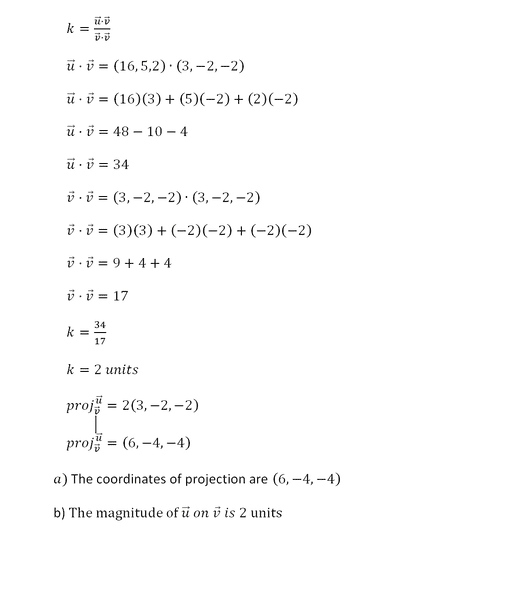# Find the Coordinates/Magnitude of projection of two vectors

## Homework Statement

Given two vectors u→=(16,5,2) and v→=(3,−2,−2) find the following:
1. The coordinates of the projection of u→ on v→

2. The magnitude of the projection of u→ on v→
.

## The Attempt at a Solution

I have never done a question like this before, I was curious did I do it correctly? If not should I instead find the projection from point A to B?
Here's my attempt: (I wrote it out in word to make it easier to read)#### Attachments

Last edited:

andrewkirk
Homework Helper
Gold Member
(a) is correct. For (b) you need to work out the magnitude of the vector that is the answer to (a). Square each component, add, then take the square root. The answer will be more than 2.

•Physics345
(a) is correct. For (b) you need to work out the magnitude of the vector that is the answer to (a). Square each component, add, then take the square root. The answer will be more than 2.
Oh wow That's all they want me to do I've been doing that I assumed this was asking me to do something else because it's asking for "Magnitude of the projection". I assumed it was different. Anyways I appreciate it, thanks for pointing that out to me.

=√(6)^2 +(-4)^2 +(-4)^2
=√72

Therefore,the magnitude of u ⃗ on v ⃗ is √72

Thanks again =)